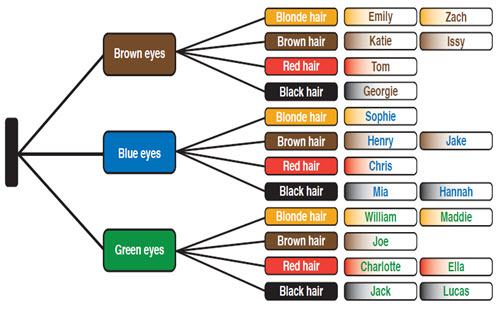Go to website

# reSolve: Multiplication: Cartesian Product

This sequence of two lessons introduces the idea of multiplication as a Cartesian product, using the language of 'for each'. Students learn to use a tree diagram to find the number of possible combinations that can be made in an animal mix-and-match book. They learn how a simpler problem can be used to help solve a larger, more complex problem. Students then design an avatar that has two or three different features and work as a class to find the total number of possible avatars. They look at how all combinations can be represented as an array and learn how to create a tree diagram. Each lesson is outlined in detail including curriculum links, vocabulary, materials needed, sample answers, discussion points and student resources. This sequence is part of the reSolve: Mathematics by Inquiry program.

Year level(s) Year 4
Audience Teacher
Purpose Teaching resource
Teaching strategies and pedagogical approaches Mathematics investigation
Keywords division, combinations, Cartesian product, multiplication

## Curriculum alignment

Curriculum connections Numeracy
Strand and focus Number, Build understanding, Apply understanding
Topics Multiplication and division
AC: Mathematics content descriptions
ACMNA075 Recall multiplication facts up to 10 × 10 and related division facts
ACMNA076 Develop efficient mental and written strategies and use appropriate digital technologies for multiplication and for division where there is no remainder
National numeracy learning progression Multiplicative strategies - MuS5, MuS6